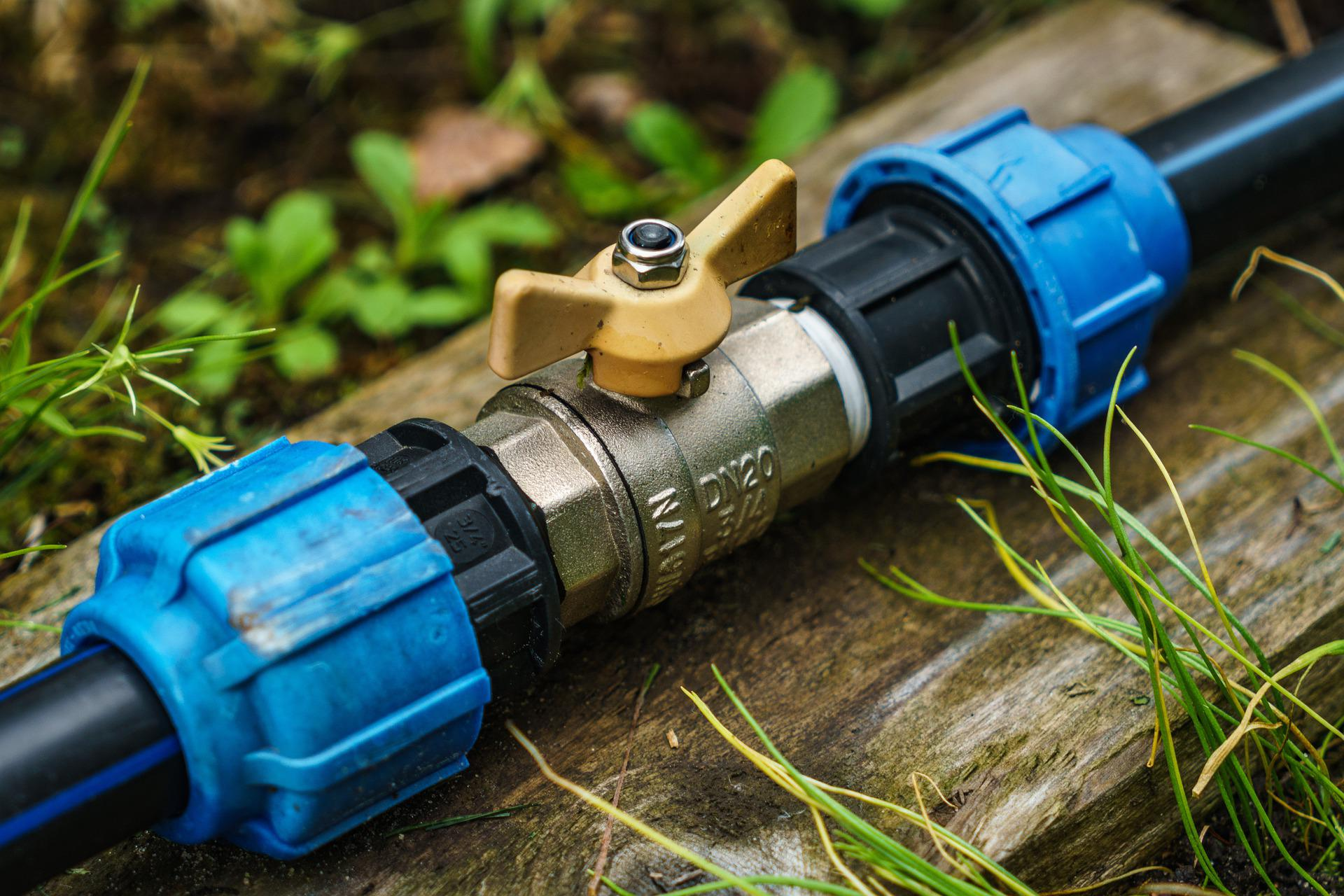# How to Check if a Horizontal Pipe Carrying Liquid is Full

In this post I want to share how to check if a horizontal pipe carrying liquid is full or partially full. We can use the following criteria to check:

• The pipe is full if: Q/d5 ≥ 10.2
• The pipe is partially full if: Q/d5 < 10.2

For partially full pipe, we need to do partially full flow analysis as follows.

x = ln (Q/d2.5)

Then we calculate height of liquid in the pipe using the following equation:

H/D = 0.446 + 0.272x + 0.0397x2 – 0.0153x3 – 0.003575x4

Equivalent diameter (De) is calculated by the following equation:

De/D = -0.01130 + 3.040 (H/D) – 3.461 (H/D)2 + 4.108 (H/D)3 – 2.638 (H/D)4

Where:

D = pipe diameter, ft

De = equivalent diameter, ft

H = height of liquid in the pipe, ft

Q = flow rate, gpm

d = pipe diameter, in

q = flow rate, ft/s

u = velocity, ft/s

De is substituted for D in subsequent flow analysis.

I made a simple spreadsheet to check pipe carrying liquid is full or partially full, what the liquid height is, and pipe equivalent diameter. Please check and download if you find this useful.